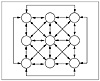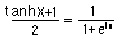# 113.C++ Neural Networks and Fuzzy Logic by Valluru B. Rao M&T Books, IDG Books Worldwide, Inc. ISBN: 1558515526   Pub Date: 06/01/95

### Neural Network for Traveling Salesperson Problem

Hopfield and Tank used a neural network to solve a traveling salesperson problem. The solution you get may not be optimal in certain instances. But by and large you may get a solution close to an optimal solution. One cannot find for the traveling salesperson problem a consistently efficient method of solution, as it has been proved to belong to a set of problems called NP-complete problems. NP-complete problems have the distinction that there is no known algorithm that is efficient and practical, and there is little likelihood that such an algorithm will be developed in the future. This is a caveat to keep in mind when using a neural network to solve a traveling salesperson problem.

#### Network Choice and Layout

We will describe the use of a Hopfield network to attempt to solve the traveling salesperson problem. There are n2 neurons in the network arranged in a two-dimensional array of n neurons per row and n per column. The network is fully connected. The connections in the network in each row and in each column are lateral connections. The layout of the neurons in the network with their connections is shown in Figure 15.1 for the case of three cities, for illustration. To avoid cluttering, the connections between diagonally opposite neurons are not shown.Figure 15.1  Layout of a Hopfield network for the traveling salesperson problem.

The most important task on hand then is finding an appropriate connection weight matrix. It is constructed taking into account that nonvalid tours should be prevented and valid tours should be preferred. A consideration in this regard is, for example, no two neurons in the same row (column) should fire in the same cycle of operation of the network. As a consequence, the lateral connections should be for inhibition and not for excitation.

In this context, the Kronecker delta function is used to facilitate simple notation. The Kronecker delta function has two arguments, which are given usually as subscripts of the symbol δ. By definition δik has value 1 if i = k, and 0 if ik. That is, the two subscripts should agree for the Kronecker delta to have value 1. Otherwise, its value is 0.

We refer to the neurons with two subscripts, one for the city it refers to, and the other for the order of that city in the tour. Therefore, an element of the weight matrix for a connection between two neurons needs to have four subscripts, with a comma after two of the subscripts. An example is wik,lj referring to the weight on the connection between the (ik) neuron and the (lj) neuron. The value of this weight is set as follows:

Wik,lj = -A1δil(1-δkj)-A2δkj(1-δkj(1-δil)-A3-A4 dilj, k+1 + δj,k-1)

Here the negative signs indicate inhibition through the lateral connections in a row or column. The -A3 is a term for global inhibition.

#### Inputs

The inputs to the network are chosen arbitrarily. If as a consequence of the choice of the inputs, the activations work out to give outputs that add up to the number of cities, an initial solution for the problem, a legal tour, will result. A problem may also arise that the network will get stuck at a local minimum. To avoid such an occurrence, random noise can be added. Usually you take as the input at each neuron a constant times the number of cities and adjust this adding a random number, which can differ for different neurons.

#### Activations, Outputs, and Their Updating

We denote the activation of the neuron in the ith row and jth column by aij, and the output is denoted by xij. A time constant τ, and a gain λ are used as well. A constant m is another parameter used. Also, Δt denotes the increment in time, from one cycle to the next. Keep in mind that the index for the summation Σ ranges from 1 to n, the number of cities. Excluded values of the index are shown by the use of the symbol ≠.

The change in the activation is then given by Δaij, where:

Δaij = Δt (Term1 + Term2 + Term3 + Term4 + Term5)
Term1 = - aij
Term2 = - A1 k≠jxik
Term3 = - A2
Σk≠ixkj
Term4 = - A3i Σkxik - m)
Term5 = - A4 Σk≠idik(xk,j+1 + xk,j-1)

To update the activation of the neuron in the ith row and jth column, you take:

aijnew = aijold + Δaij

The output of a neuron in the ith row and jth column is calculated from:

xin = (1 + tanh(λaij))/2

NOTE:, which is the original sigmoid function

The function used here is the hyperbolic tangent function. The gain parameter mentioned earlier λ is. The output of each neuron is calculated after updating the activation. Ideally, you want to get the outputs as 0’s and 1’s, preferably a single one for each row and each column, to represent a tour that satisfies the conditions of the problem. But the hyperbolic tangent function gives a real number, and you have to settle for a close enough value to 1 or 0. You may get, for example, 0.96 instead of 1, or 0.07 instead of 0. The solution is to be obtained from such values by rounding up or down so that 1 or 0 will be used, as the case may beC++ Neural Networks and Fuzzy Logic
ISBN: 1558515526
EAN: 2147483647
Year: 1995
Pages: 139

Similar book on Amazon